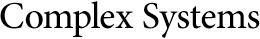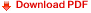## Evaluating the Complexity of Mathematical Problems: Part 1Cristian S. Calude
University of Auckland, New Zealand
www.cs.auckland.ac.nz/~cristian

Elena Calude
Massey University at Albany, New Zealand
www.massey.ac.nz/~ecalude

#### Abstract

In this paper we provide a computational method for evaluating the complexity of a large class of mathematical problems in a uniform way. The method, which is inspired by A New Kind of Science , is based on the possibility of completely describing complex mathematical problems, such as the Riemann hypothesis, in terms of very simple programs. The method is illustrated on a variety of examples from different areas of mathematics and its power and limits are studied.

https://doi.org/10.25088/ComplexSystems.18.3.267